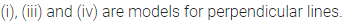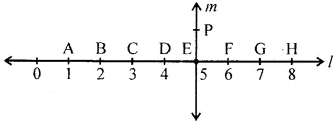ML Aggarwal Class 6 Solutions Chapter 11 Understanding Symmetrical Shapes Ex 11.3 for ICSE Understanding Mathematics acts as the best resource during your learning and helps you score well in your exams.

## ML Aggarwal Class 6 Solutions for ICSE Maths Chapter 11 Understanding Symmetrical Shapes Ex 11.3

Question 1.
Which of the following are models for perpendicular lines?
(i) The adjacent edges of a postcard.
(ii) the lines of a railway track.
(iii) The line segment forming the letter ‘L’.
(v) The line segments forming the letter ‘V’.
Solution:Question 2.
In the figure given below, line l is perpendicular to line m.(a) Is CE = EG?
(b) Does $$\overleftrightarrow { PE }$$ bisect segment $$\overline{\mathrm{BH}}$$ ?
(c) Identify any two line segments for which $$\overleftrightarrow { PE }$$ is the perpendicular bisector.
(d) Are these true?
(i) AC > FG
(ii) CD = GH
(iii) BC < EG.
Solution: